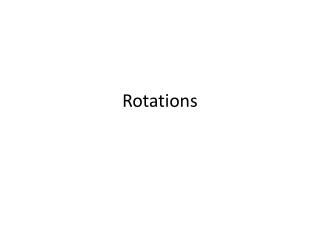# Rotations - PowerPoint PPT PresentationDownload PresentationRotations

RotationsDownload Presentation## Rotations

- - - - - - - - - - - - - - - - - - - - - - - - - - - E N D - - - - - - - - - - - - - - - - - - - - - - - - - - -
##### Presentation Transcript

1. Rotations

2. Two more days until the Unit Test!!! • Take out your homework from last night. • Take out a piece of graph paper. • Title it 12.3: Rotations

3. 12.3: Rotations • Learning Objective: • SWBAT identify and draw rotations.

4. Rotation • Rotation • Transformation that turns a figure around a fixed point, called the center of rotation. • Each point and its image are the same distance from P • all the angles with vertex P formed by a point and its image are congruent

5. Isometry • If I say that a rotated image is isometric to it’s pre-image, what do you think I mean? • Think-pair-share • Isometry • image of a rotated figure is congruentto the pre-image. • This is also known as a rigid motion

6. Rigid Motion • A transformation that is isometric • Which transformation is NOT a rigid motion? • Translation • Reflection • Rotation • Dilation

7. Whiteboards Tell whether each transformation appears to be a rotation. Explain. B. A. No; the figure appears to be flipped. Yes; the figure appears to be turned around a point.

8. Whiteboards Tell whether each transformation appears to be a rotation. b. a. Yes, the figure appears to be turned around a point. No, the figure appears to be a translation.

9. Drawing Rotations • Materials: • Patty paper • Pencil • Straight edge • Protractor • One paper per group divided into fourths

10. Steps • On your sheet of paper draw a triangle and a point. The point will be the center of rotation. • Place a sheet of patty paper on top of the diagram. Trace the triangle and the point. Label it pre-image. • Hold your pencil down on the point and rotate the bottom paper counterclockwise. • Trace the triangle. Label it image.

11. Draw a segment from each vertex of your pre-image and image to the center of rotation. • What do you notice about distance from the center of rotation to your pre-image and image? • How can you figure out how many degrees your figure was rotated?

12. A reflection is what type of rotation? • Think-pair-share. • Use this image to help you think about it:

13. Rotating 180 About the Origin • Rotate ΔJKL with vertices J(2, 2), K(4, –5), and L(–1, 6) by 180° about the origin. • What is the rule? • (x,y) (-x, -y) • Use arrow notation and then plot the pre-image and the image.

14. (x,y) (-x, -y)

15. By 90 About the Origin • Rotate A(2, -1), B (4, 1) and C (3,3) by 90 degrees about the origin ( counterclockwise) • What is the rule? • (x,y) (-y, x) • First do arrow notation • Then graph the pre-image and the image

16. Check your work!

17. Graphing Whiteboards • Rotate A(2, -1), B (4, 1) and C (3,3) by 180 degrees about the origin • First what is the rule? • Graph the pre image • Graph the image

18. Math Joke of the Day • Doctor: How did you get that sprain? • Patient: It was a bad rotation. Credit to Period 1

19. Closure 1. Tell whether the transformation appears to be a rotation. yes

20. Closure Rotate ∆RST with vertices R(–1, 4), S(2, 1), and T(3, –3) about the origin by the given angle. 3. 90° R’(–4, –1), S’(–1, 2), T’(3, 3) 4. 180° R’(1, –4), S’(–2, –1), T’(–3, 3)

21. Homework • Work on the study guide from my website • Pg 842: # 1-10 ( skip 5, 6), 14, 27- 30